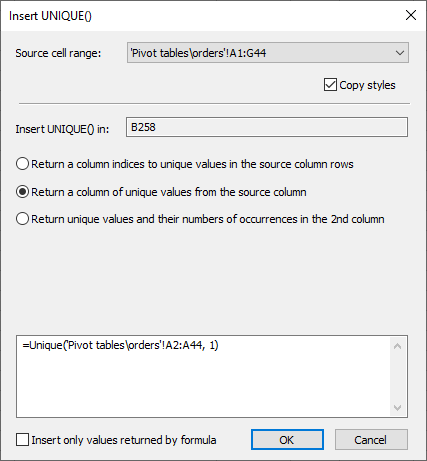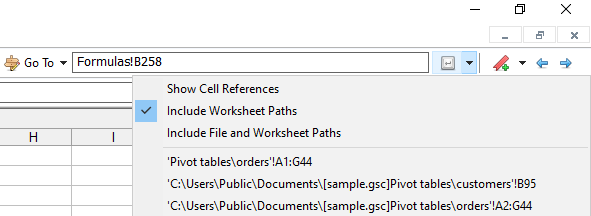Unique() function

Unique(vector, [type])

Searches a column (array or range) for unique values.

If 'type' is 0, the 'unique' function returns a vector of indices to the subsequent unique values found in 'vector'.

If 'type' is 1, the 'unique' function returns a vector of unique values found in 'vector'.

If 'type' is 2, the 'unique' function returns a two-column array.
The first column contains unique values, the second one: the number of occurrences of the corresponding value.

If 'type' is omitted, it's assumed to be 1.

Unique() examples:

=unique({2; 3; 1; 2; 5; 3; 1}, 1) returns {1; 2; 3; 5}

=unique({"a"; "b"; "cd"; "a"; "cd"; "b"; "d"}, 0) returns {1; 2; 3; 5}

=unique({"a"; "b"; "cd"; "a"; "cd"; "b"; "d"}, 2) returns { "a", 2; "b", 2; "cd", 2; "d", 1}

You can insert the Unique() function with just two clicks using the Insert > UNIQUE() command. The displayed "Insert UNIQUE()" dialog box determines the optimum parameters including the option to copy styles from the selected source data range and automatically creates the formula.The source range 16 element list is a global list created and stored in the settings file. Subsequent source ranges are added in a circular manner by clicking one of the following:

The "Copy as Location" menu commandThe "Enter" toolbar button